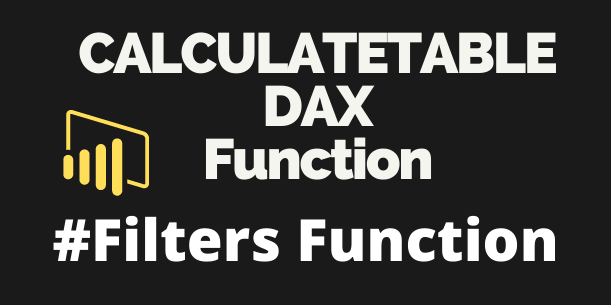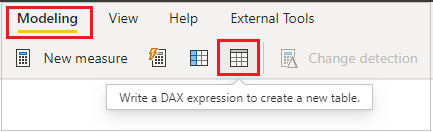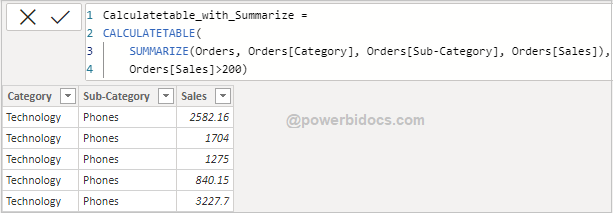Home » DAX » DAX CALCULATETABLE Function

# DAX CALCULATETABLE FunctionCALCULATETABLE DAX function comes under Power BI Filter DAX category and It evaluates a table expression in a context modified by the given filters. It returns a table of values.

CALCULATETABLE allows you to create virtual tables that you can filter using multiple conditions and use that table to make further calculations.

### Syntax

`CALCULATETABLE(<expression> , <filter1>  , <filter2> , …)`

### Parameters

expression – The table expression to be evaluated.

filter1, filter2… –  (Optional) Boolean expressions or table expressions that defines filters, or filter modifier functions.

### Let’s get started-

Download Sample dataset- Global_superstore_2016.xls

Let’s understand behavior of CALCULATETABLE function, following these steps-

Step-1: Go to Modeling Tab > Select “DAX expression to create a new table”.Create table

Step-2: After that Write below DAX functionCalculatetable dax

Step-3: As you can see in below screenshot, it return new table with given condition data where sales is > 200.Calculatetable dax result

### CALCULATETABLE with Measure-

You can also use CALCULATETABLE with measure based on your requirement. It will create virtual tables that you can filter using multiple conditions and use that table to make further calculations.

Step-1: Create one measure and write below DAX code.

```Calculate_Table_with_measure =

SUMX(

CALCULATETABLE(Orders, Orders[Sales]>200),

Orders[Sales])```Calculate table with measure

Step-2: Add one card visual and drag measure.Calculatetable measure output

### CALCULATETABLE function with SUMMARIZE function

As you have seen above, a CALCULATETABLE function returns a table based on given filter condition, and it return all columns of base table.

But if you want to see only specific columns then you can use SUMMARIZE DAX function with CALCULATETABLE.

Now go to modeling tab > Add new DAX table

```Calculatetable_with_Summarize =

CALCULATETABLE(

SUMMARIZE(Orders, Orders[Category], Orders[Sub-Category], Orders[Sales]),

Orders[Sales]>200)```

See the output-Output Calculatetable with Summarize

Refer DAX- SUMMARIZE

Hope you enjoyed the post. Your valuable feedback, question, or comments about this post are always welcome or you can leave us message on our contact form , we will revert to you asap.